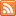# Content Tagged “LO 6.20”## Summary (Unit 3B – Random Variables)

Published: Jan 20th, 2014

Random Variables Binomial Random Variables Continuous Random Variables We have almost reached the end our discussion of probability. We were introduced to the important concept of random variables, which are quantitative variables whose […]## Normal Applications

Published: Feb 9th, 2013

Working with Non-standard Normal Values – Finding Probabilities Finding Normal Scores – Backwards! Given probability, find the score. Normal Approximation for Binomial Rule of Thumb Continuity Correction CO-6: Apply basic concepts of probability, […]## Standard Normal Distribution

Published: Feb 8th, 2013

Finding Probabilities for a Normal Random Variable Standardizing Values Finding Probabilities with the Normal Calculator and Table Finding Standard Normal Scores CO-6: Apply basic concepts of probability, random variation, and commonly […]